# Equations and Inequalities Unit 8 Solving Inequalities Vocabulary

• Slides: 12Equations and Inequalities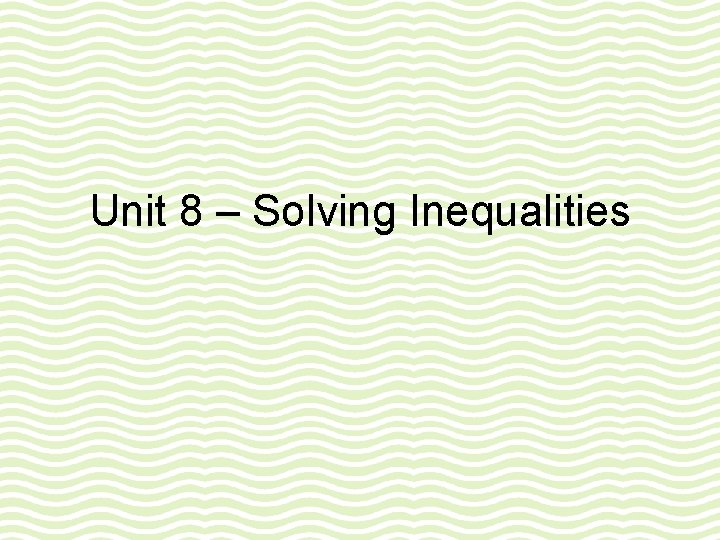Unit 8 – Solving Inequalities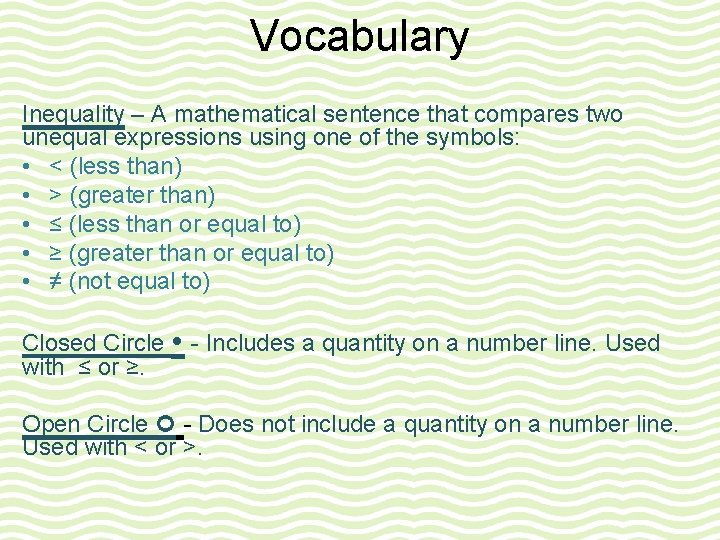Vocabulary Inequality – A mathematical sentence that compares two unequal expressions using one of the symbols: • < (less than) • > (greater than) • ≤ (less than or equal to) • ≥ (greater than or equal to) • ≠ (not equal to) Closed Circle - Includes a quantity on a number line. Used with ≤ or ≥. Open Circle - Does not include a quantity on a number line. Used with < or >.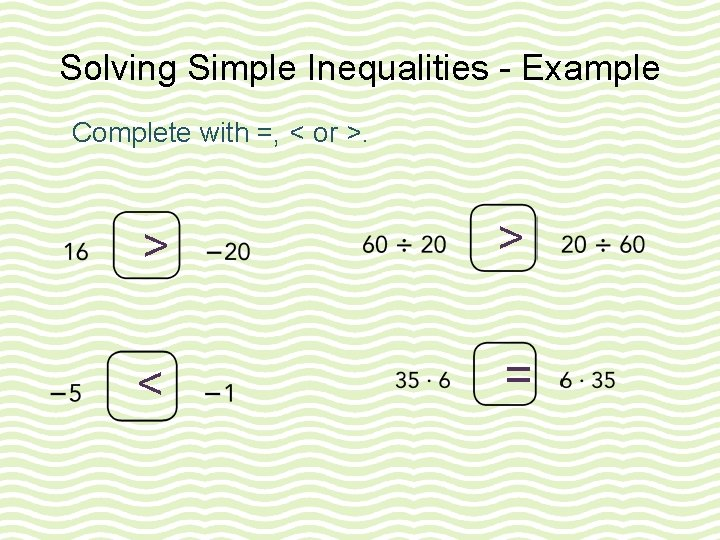Solving Simple Inequalities - Example Complete with =, < or >. > > < =Determining Solutions for Inequalities Example Determine 4 solutions for each inequality. Then represent the solutions of each inequality on a number line. y >7 When y = _____, y > 7 is true. The inequality y > 7 is true for any value of y that is greater than 7.Determining Solutions for Inequalities Example Determine 4 solutions for each inequality. Then represent the solutions of each inequality on a number line. m < 28 When m = _____, m < 28 is true. The inequality m < 28 is true for any value of m that is less than 28.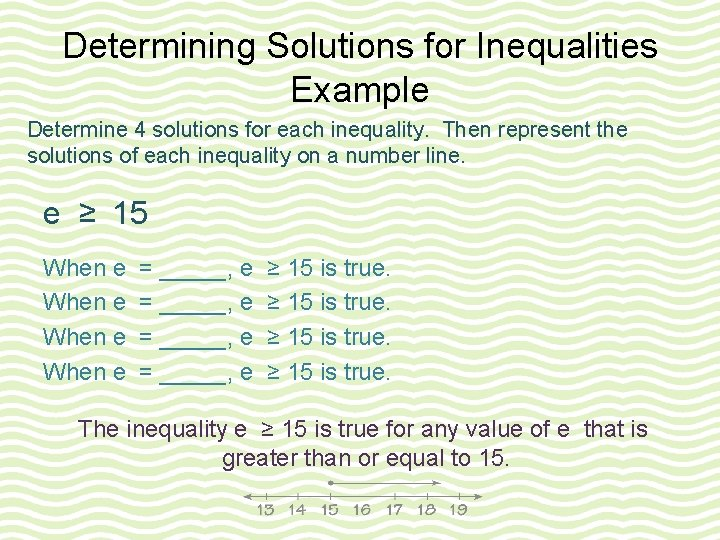Determining Solutions for Inequalities Example Determine 4 solutions for each inequality. Then represent the solutions of each inequality on a number line. e ≥ 15 When e = _____, e ≥ 15 is true. The inequality e ≥ 15 is true for any value of e that is greater than or equal to 15.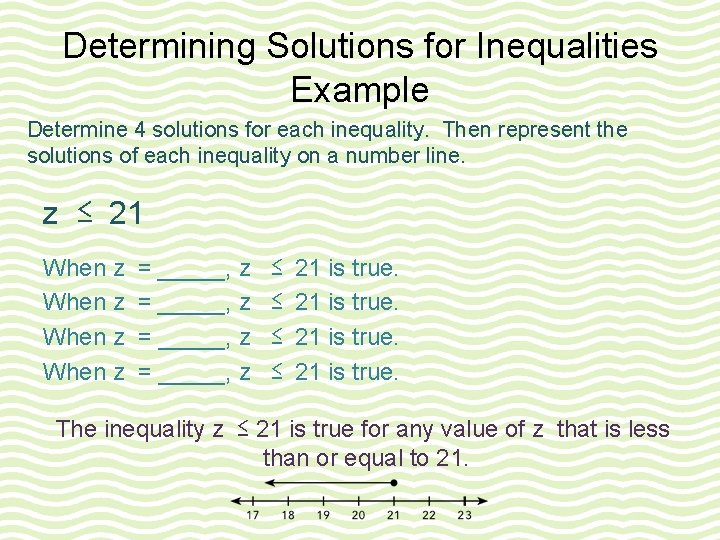Determining Solutions for Inequalities Example Determine 4 solutions for each inequality. Then represent the solutions of each inequality on a number line. z ≤ 21 When z = _____, z ≤ ≤ 21 is true. The inequality z ≤ 21 is true for any value of z that is less than or equal to 21.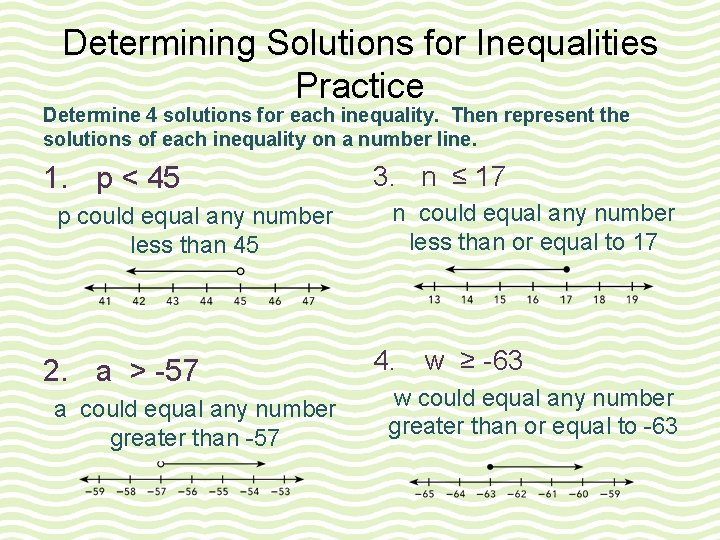Determining Solutions for Inequalities Practice Determine 4 solutions for each inequality. Then represent the solutions of each inequality on a number line. 1. p < 45 p could equal any number less than 45 2. a > -57 a could equal any number greater than -57 3. n ≤ 17 n could equal any number less than or equal to 17 4. w ≥ -63 w could equal any number greater than or equal to -63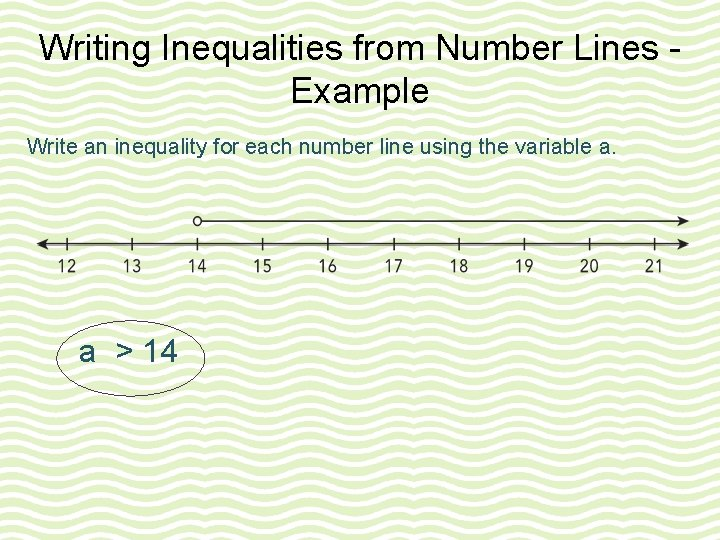Writing Inequalities from Number Lines Example Write an inequality for each number line using the variable a. a > 14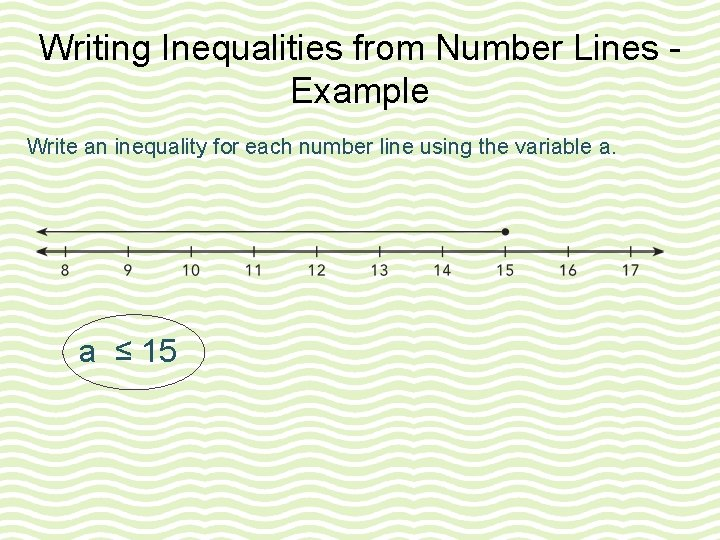Writing Inequalities from Number Lines Example Write an inequality for each number line using the variable a. a ≤ 15Writing Inequalities from Number Lines Practice Write an inequality for each number line using the variable a. a ≥ 11 a < 14